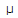# Electronics - Capacitors

### Exercise :: Capacitors - General Questions

36.

What is the capacitive reactance if I = 1 amp, V = 2 volts, C = 5 F, and frequency = 10 Hz?

 A. 0.2B. 0.5C. 0.02D. 0.003Explanation:

No answer description available for this question. Let us discuss.

37.

What is wrong with a leaky capacitor?

 A. It is open. B. It is shorted. C. The dielectric resistance has increased. D. The dielectric resistance has decreased.

Explanation:

No answer description available for this question. Let us discuss.

38.

What is the value of a capacitor labeled 102?

 A. 10,000 pF B. 1000 pF C. 1000F D. 10,000F

Explanation:

No answer description available for this question. Let us discuss.

39.

If the dielectric constant of a capacitor is decreased, then its capacitance will:

 A. increase B. decrease C. remain the same D. be destroyed

Explanation:

No answer description available for this question. Let us discuss.

40.

What is the dielectric constant for ceramic?

 A. between 6 and 8000 B. 5.0 C. between 5 and 1000 D. 3000